A ball is thrown upward with a certain speed. It passes through the same point at 3 second and 7 second from the start. The maximum height achieved by the ball is

1.  500 m

2.  250 m

3.  125 m

4.  450 m

Concept Questions :-

Uniformly accelerated motion
High Yielding Test Series + Question Bank - NEET 2020

Difficulty Level:

For the following acceleration versus time graph the corresponding velocity versus displacement graph is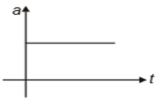1.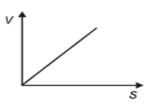2.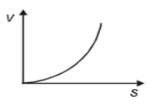3.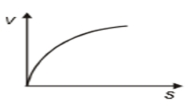4.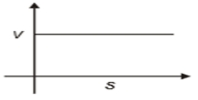Concept Questions :-

Uniformly accelerated motion
High Yielding Test Series + Question Bank - NEET 2020

Difficulty Level:

A stone falls freely from rest from a height h and it travels a distance $\frac{9h}{25}$ in the last second. The value of h is:

1. 145 m

2. 100 m

3. 122.5 m

4. 200 ms

Concept Questions :-

Uniformly accelerated motion
High Yielding Test Series + Question Bank - NEET 2020

Difficulty Level:

A particle is thrown upwards from ground. It experiences a constant resistance force which can produce retardation of 2 $m/{s}^{2}$. The ratio of time of ascent to the time of descent is:

(1) 1:1

(2) $\sqrt{\frac{2}{3}}$

(3) $\frac{2}{3}$

(4) $\sqrt{\frac{3}{2}}$

Concept Questions :-

Uniformly accelerated motion
High Yielding Test Series + Question Bank - NEET 2020

Difficulty Level:

A bullet loses $\frac{1}{20}$ of its velocity passing through a plank. The least number of planks required to stop the bullet is (All planks offers same retardation)

(1) 10

(2) 11

(3) 12

(4) 23

Concept Questions :-

Uniformly accelerated motion
High Yielding Test Series + Question Bank - NEET 2020

Difficulty Level:

A point moves with uniform acceleration and v1, v2 and v3 denote the average velocities in the three successive intervals of time t1, t2 and t3. Which of the following relations is correct ?

(1) $\left({v}_{1}-{v}_{2}\right):\left({v}_{2}-{v}_{3}\right)=\left({t}_{1}-{t}_{2}\right):\left({t}_{2}+{t}_{3}\right)$

(2) $\left({v}_{1}-{v}_{2}\right):\left({v}_{2}-{v}_{3}\right)=\left({t}_{1}+{t}_{2}\right):\left({t}_{2}+{t}_{3}\right)$

(3) $\left({v}_{1}-{v}_{2}\right):\left({v}_{2}-{v}_{3}\right)=\left({t}_{1}-{t}_{2}\right):\left({t}_{1}-{t}_{3}\right)$

(4) $\left({v}_{1}-{v}_{2}\right):\left({v}_{2}-{v}_{3}\right)=\left({t}_{1}-{t}_{2}\right):\left({t}_{2}-{t}_{3}\right)$

Concept Questions :-

Uniformly accelerated motion
High Yielding Test Series + Question Bank - NEET 2020

Difficulty Level:

The initial velocity of the particle is 10 m/sec and its retardation is 2 m/sec2. The distance moved by the particle in 5th second of its motion is

(1) 1 m

(2) 19 m

(3) 50 m

(4) 75 m

Concept Questions :-

Uniformly accelerated motion
High Yielding Test Series + Question Bank - NEET 2020

Difficulty Level:

A motor car moving with a uniform speed of 20 m/sec comes to stop on the application of brakes after travelling a distance of 10 m Its acceleration is

(1) 20 m/sec2

(2) –20 m/sec2

(3) –40 m/sec2

(4) +2 m/sec2

Concept Questions :-

Uniformly accelerated motion
High Yielding Test Series + Question Bank - NEET 2020

Difficulty Level:

The velocity of a body moving with a uniform acceleration of 2 m/sec2 is 10 m/sec. Its velocity after an interval of 4 sec is

(1) 12 m/sec

(2) 14 m/sec

(3) 16 m/sec

(4) 18 m/sec

Concept Questions :-

Uniformly accelerated motion
High Yielding Test Series + Question Bank - NEET 2020

Difficulty Level:

A particle starting from rest moving with constant acceleration travels a distance x in first 2 seconds and a distance y in next two seconds, then

(1) y = x

(2) y = 2x

(3) y = 3x

(4) y = 4x

Concept Questions :-

Uniformly accelerated motion